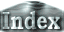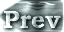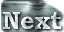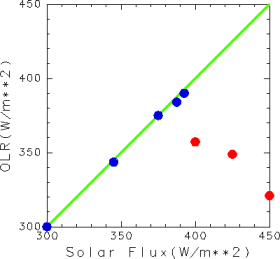# 3. Results### b. The Value of the 3D Runaway Limit

 In order to obtain critical value of solar constant at which the thermally runaway state emerges, the global mean incident solar flux and global mean OLR for all experiments is plotted in Figure 1. The values of OLR shown in the plot are the time-averaged global mean values from Day 950 to Day 1000, except for the S1600 experiment (in which the global mean values of incident solar radiation flux are 400 W/m2). For S1600, the value is the time-averaged value from Day 1950 to Day 2000. When the value of solar constant is 1570 W/m2 (global mean incident solar influx is 392.5 W/m2) or less, OLR is almost equal to the incident solar radiation flux and is regarded as having reached an equilibrium value. In contrast, when solar constant is 1600 W/m2 (the global mean incident solar influx is 400 W/m2 ) or more, OLR falls below 350 W/m2. In experiments S1600 and S1700, the values of OLR gradually decrease with time, whereas surface temperature increases with time (not shown in plot). In all cases where S ≥ 1600 W/m2, the system failed to reach an equilibrium state and the thermal runaway state emerged. Therefore, it can be concluded that the solar constant at which the thermal runaway state emerges (runaway limit) is slightly below 1600 W/m2.Figure 1: Global mean incident solar flux and global mean OLR for all experiments (W/m2). The blue points show the results in which the system reaches an equilibrium state and the red points show those in which the thermally runaway state emerges. Note that in all cases where S ≥ 1600 W/m2 the system failed to reach an equilibrium state. As a result, the values of OLR of these case shown in this figure do not have physical meaning, because OLR gradually decreases with time. For experiment S1600, the value at Day 2000 is used to show a clearly the runaway limit value. To view the results of all experiments, click the corresponding blue and red points on the figure (Appendix G).

### 3.b. The Value of the 3D Runaway Limit